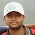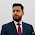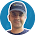## Pages

SUBSCRIBE HERE TO GET POST UPDATES VIA EMAIL :

Delivered by FeedBurner

# How To Extract Table Data/Read Table Data Using Selenium WebDriver Example

How To Get Number Of Rows Using Selenium WebDriver

Look at the firebug view of above HTML table. In any web table, Number of <tr> tags Inside <tbody> tag describes the number of rows of table. So you need to calculate that there are how many <tr> tags Inside <tbody> tag. To get row count for above given example table, we can use webdriver statement like bellow.

``int Row_count = driver.findElements(By.xpath("//*[@id='post-body-6522850981930750493']/div/table/tbody/tr")).size();``

In above syntax, .size() method with webdriver's findElements method will retrieve the count of <tr> tags and store It In Row_count variable.

How To Get Number Of Columns Using Selenium WebDriver

Same way In any web table, Number of <td> tags from any <tr> tag describes the number of columns of table as shown In bellow given Image.

So for getting number of columns, we can use syntax like bellow.
``int Col_count = driver.findElements(By.xpath("//*[@id='post-body-6522850981930750493']/div/table/tbody/tr/td")).size();``

Now we have row count and column count. One more thing you require to read data from table Is generating Xpath for each cell of column. Look at bellow given xpath syntax of 1st cell's 1st and 2nd rows.

//*[@id='post-body-6522850981930750493']/div/table/tbody/tr/td
//*[@id='post-body-6522850981930750493']/div/table/tbody/tr/td

Highlighted string Is common for both cell's xpath. Only changing Is row number Inside tr node. So here we can pass Row_count variable's value  Inside tr node.

Same way, look at bellow given xpath syntax of 1st and 2nd cells of 1st row. Highlighted string Is common for both cell's xpath. Only changing Is column number Inside td node. So here we can pass Col_count variable's value Inside td node.

//*[@id='post-body-6522850981930750493']/div/table/tbody/tr/td
//*[@id='post-body-6522850981930750493']/div/table/tbody/tr/td

All above thing Is applied In bellow given example. Simply run It In your eclipse using testng and observe output In console.
``````package Testng_Pack;

import java.util.concurrent.TimeUnit;

import org.openqa.selenium.By;
import org.openqa.selenium.WebDriver;
import org.openqa.selenium.firefox.FirefoxDriver;
import org.testng.annotations.AfterTest;
import org.testng.annotations.BeforeTest;
import org.testng.annotations.Test;

public class table {

WebDriver driver = null;
@BeforeTest
public void setup() throws Exception {
System.setProperty("webdriver.gecko.driver", "D:\\Selenium Files\\geckodriver.exe");
driver = new FirefoxDriver();
driver.manage().window().maximize();
driver.manage().timeouts().implicitlyWait(15, TimeUnit.SECONDS);
driver.get("http://only-testing-blog.blogspot.in/2013/09/test.html");
}

@AfterTest
public void tearDown() throws Exception {
driver.quit();
}

@Test
public void print_data(){

//Get number of rows In table.
int Row_count = driver.findElements(By.xpath("//*[@id='post-body-6522850981930750493']/div/table/tbody/tr")).size();
System.out.println("Number Of Rows = "+Row_count);

//Get number of columns In table.
int Col_count = driver.findElements(By.xpath("//*[@id='post-body-6522850981930750493']/div/table/tbody/tr/td")).size();
System.out.println("Number Of Columns = "+Col_count);

//divided xpath In three parts to pass Row_count and Col_count values.
String first_part = "//*[@id='post-body-6522850981930750493']/div/table/tbody/tr[";
String second_part = "]/td[";
String third_part = "]";

//Used for loop for number of rows.
for (int i=1; i<=Row_count; i++){
//Used for loop for number of columns.
for(int j=1; j<=Col_count; j++){
//Prepared final xpath of specific cell as per values of i and j.
String final_xpath = first_part+i+second_part+j+third_part;
//Will retrieve value from located cell and print It.
String Table_data = driver.findElement(By.xpath(final_xpath)).getText();
System.out.print(Table_data +"  ");
}
System.out.println("");
System.out.println("");
}
}
}``````

Console output of above given example will looks like bellow.
``````Number Of Rows = 3
Number Of Columns = 6
11  12  13  14  15  16

21  22  23  24  25  26

31  32  33  34  35  36``````
Here you can see, all the values of table are extracted and printed.

1.It's nice, But the problem starts here after reading data, i want to validate column's data.
example: if i want to validate column 2 (12,22,32) then how??

2.Want to read & write data from datagrid in specific date range using java & selenium webdriver ,below mention URL please guide me how to pick data in specific date range .

http://www.nasdaq.com/symbol/ab/institutional-holdings

3.it is a bit late due to the post to give a question: but I shall try anyway.
First off love the tutorial was a good insight for a beginner. but this solution is not applicable to a python based driver? what is the .size(); equivalent for python script?
Kind Regards....

4.Thanks allot to blog. Most useful.

5.This is good one.

6.Thanks for blog...nice explanation..

7.That's a quick overview of grid handling.
How to insert data into textarea cell having elipses button which open window having input fields and in button.

8.WebElement f=driver.findElement(By.xpath(".//*[@id='post-body-6522850981930750493']/div/table/tbody"));
List rows=f.findElements(By.tagName("tr"));
System.out.println("Number of rows="+rows.size());
List columns=f.findElements(By.tagName("td"));
System.out.println("Number of columns="+columns.size());
System.out.println(f.getText());
try this same output...

9.thanks a lots ...nice blog .... very helpful..... keep it up

10.How to assign each of the cells a variable and then do the same for another table and then compare two tables?

11.How to assign each of the cell text a unique variable and then do the same for another table in order to compare two different tables?

12.How to assign each of the cells a variable and then do the same for another table and then compare two tables?

13.i want to achieve below condition,
If column matches with number 543789 then get the string which is in next column.
can you get me?

1.if(Table_data = 543789)
{
str=rowdata.get(j+1).gettext;
}
So we are checking if it matches then we are picking the next column value @feroz